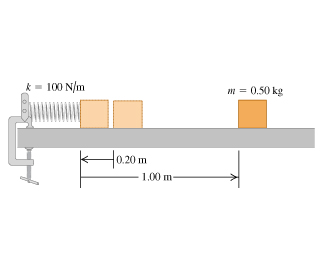# Problem: A block with mass 0.50 kg is forced against a horizontal spring of negligible mass, compressing the spring a distance of 0.20 m (Figure 1). When released, the block moves on a horizontal tabletop for 1.00 m before coming to rest. The spring constant k is 100 N/m. What is the coefficient of kinetic friction between the block and the tabletop?

###### FREE Expert Solution

Elastic potential energy:

$\overline{){\mathbf{U}}{\mathbf{=}}\frac{\mathbf{1}}{\mathbf{2}}{\mathbf{k}}{{\mathbf{x}}}^{{\mathbf{2}}}}$

Word done by a constant force:

$\overline{){\mathbf{W}}{\mathbf{=}}{\mathbf{F}}{\mathbf{d}}}$

Friction force:

$\overline{){\mathbf{f}}{\mathbf{=}}{\mathbf{\mu }}{\mathbf{N}}{\mathbf{=}}{\mathbf{\mu }}{\mathbf{m}}{\mathbf{g}}}$

x = 0.20 m

d = 1.00 m

g = 9.8 m/s2###### Problem Details

A block with mass 0.50 kg is forced against a horizontal spring of negligible mass, compressing the spring a distance of 0.20 m (Figure 1). When released, the block moves on a horizontal tabletop for 1.00 m before coming to rest. The spring constant k is 100 N/m. What is the coefficient of kinetic friction between the block and the tabletop?